# Examples#

The gallery of examples below cover different ways in which calphy can be used to calculate free energies.Calculation of free energy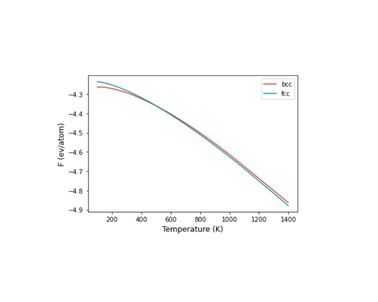Solid-solid phase transformation in Fe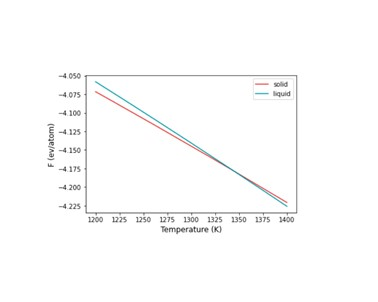Calculation of melting temperature of Cu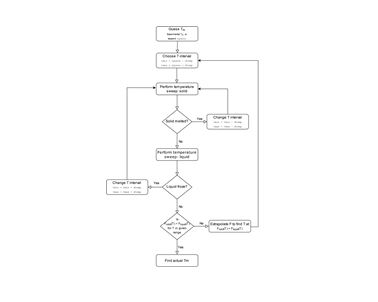Automated calculation of melting temperature of Cu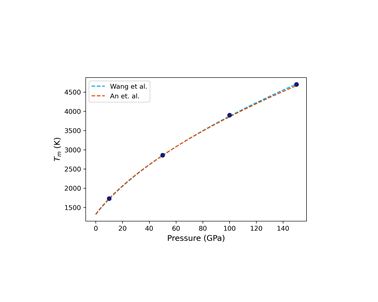Pressure-temperature phase diagram of Cu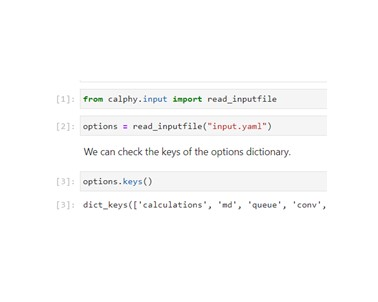Running calphy from jupyter notebooksUpsampling calculations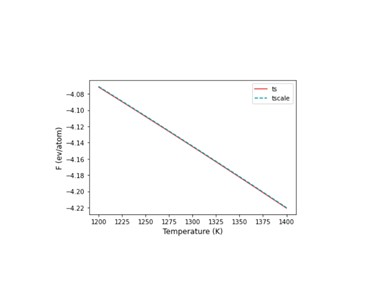Variation of free energy with temperature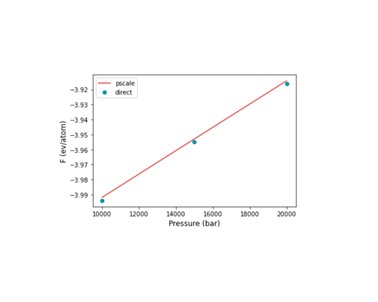Variation of free energy with pressure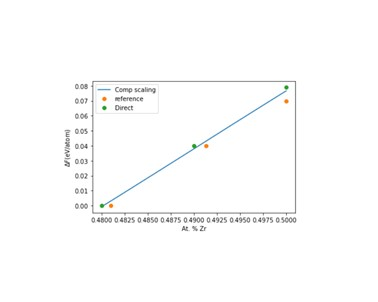Free energy with composition change in a binary systemDynamic Clausius-Clapeyron integration